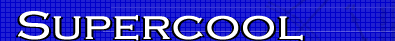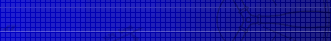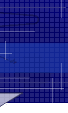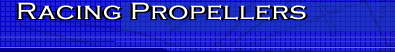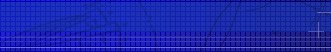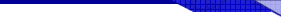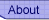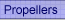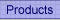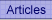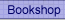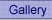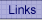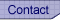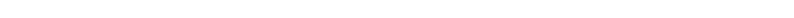﻿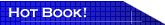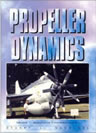Propeller Dynamics Essential reading for model aircraft contest fliers. This is the only book on the market explaining propeller theory in non-mathematical terms. A rattling good read, I know, I wrote it. Read more...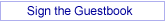## Transonic Airfoils Part 5: The Glauert-Prandtl rule.

By Joe Supercool

You must congratulate me, for this is my most outrageous title yet. In fact, is has little to do with transonic flow at all. This time we are going to look at the pure subsonic flow problem, below M = 0.7 . Well, why bother?

At the heart of propeller design is the question of how much lift we can generate efficiently for each radial station along the blade. As we have seen, the flow problem in the transonic region is little short of catastrophic. Even if the tips aren’t blowing air backwards, they can sure be soaking up engine power without giving much in return. Hence the need for special airfoil sections, which are little documented in the lay aerodynamics press.

But even for subsonic flow, there are still problems. For a start, try finding data for airfoils with low Reynolds number and Mach numbers around 0.5. We can always assign a value for the lift coefficient, but what about the zero-lift angle of these airfoils? What we need are some rules for handling the aerodynamics from M = 0 to M = .7. Luckily they exist and are quite good enough, but first let’s have some fun.

Here is a very fundamental formula for lift force, L: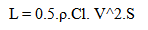First note the mixture of Greek symbols with Roman. That is a good tip-off. If it’s all in Greek characters, the author is not confused: muddled, even wrong, but not confused. If it’s all in Roman, he is uncultured. But mixed Greek and Roman? I am now going to explain this formula to you exactly as it is written.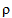is the air density. 0.5 is 0.5. Cl is a constant of proportionality called the “lift-coefficient”. Note the violation of the spelling rule “i before e except after c”. Also, Cl is not a constant, but is in fact a function of Reynolds and Mach numbers. What is more, if you look this number up in tables, you will find that it applies to a wing of infinite aspect ratio. We can live with that. V is the velocity of the airplane with the assumption that air is incompressible. In fact, air is highly compressible, but that is merely an inconvenient truth. Finally, S is the area of the infinitely long wing, so its value is the mean chord times infinity.

So we have a nice, simple formula that is about as useful, in the words of my master Andy Kerr, as tits on a bull. We shall return to this quantity Cl after a brief word from our sponsor.

I did mention a problem in finding the zero-lift angle ao of a fully subsonic airfoil. Well it turns out that there are some nice solutions to this problem based on thin airfoil theory. Abbott and Von Doenhoff give methods on page 72 attributed to Munk and separately to Pankhurst, which are quite adequate. I don’t use them, because they give the same result as a much simpler expression given by….I can’t remember! But here it is anyway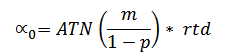where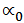= zero lift angle in degrees
m = airfoil camber … typical value .0355
p = camber line high point … typical value .4
rtd= conversion factor radians to degrees [180/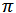}
ATN is the trigonometric operation arc tan

For these typical values, we get= -3.38 which is not too different from the value found in Soartech 8 at low Reynolds number for Clark Y.

We are nearly ready to move on. Just one more thing. There is a thing called the lift-slope. Bad English, so it must be American. When you increase the angle of attack of an airfoil, you get more lift. Surprisingly, for most thin airfoils, the value of the lift-slope is pretty well constant. That value is 0.09 degree^(-1). Good god, what?

Let’s try an example.

Choose an angle, say 5 degrees. Then the lift coefficient increases by 5 * .09 = .45 Typically, for most reasonable airfoils, the lift coefficient varies from -0.2 to 0.85. Above 0.85, the airfoil is getting close to the stall. Below -0.2, we are looking at a cambered airfoil which is about to do a negative stall!

So to review. We have a thing called lift-coefficient. Also a thing called zero-lift angle and yet another thing called lift-slope. If you have those things figured, we can move on.

What we are looking for is the behaviour of the lift coefficient as affected by Mach number. In figure 2 below, look at the red and yellow curves for Mach number less than 0.7. Mach numbers below 0.7 are generally “subsonic”. There is no point on the airfoil where the local flow has exceeded Mach 1. We are not dealing with the shock-waves which gave us the horrors in the transonic region.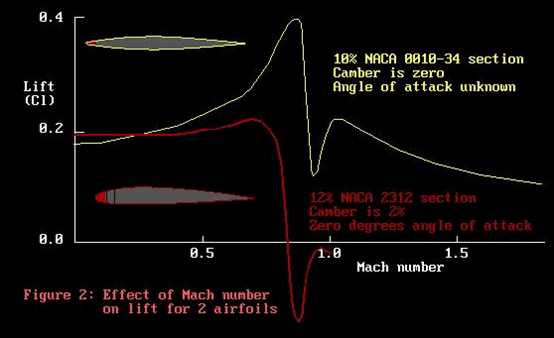Both these airfoils show an increase in the lift coefficient as the Mach number increases. There is a rather greater increase for the yellow airfoil than the red, which is confounding. For this exercise, we will pretend they are the same!
This increase in lift coefficient with Mach number is a compressibility effect. There is a very simple formula which allows us to calculate this increase in lift coefficient. The formula is called by Milne-Thompson the “Glauert correction”, and by other authors as the “Glauert-Prandtl rule”. It is of great value to propeller designers, as virtually every propeller has a strong radial change in Mach number from root to tip. In F3D and F2A, this change ranges from 0 out to .95, which is really quite a problem.

If this rule lets us cover the subsonic region, then at least we have that covered. But just what is this rule, and how may it be applied? Well, first let me have a moan. For years I have been aware of this rule, but being a simple soul I could not see how to apply it. In my defence, let me quote from some texts.

First off the rank is Godsey and Young, ”Gas Turbines for Aircraft”, 1949. Try this for a mouthful.

“Consider a flow with free-stream Mach number Mo over a thin airfoil of thickness t set at an angle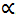. The drag and lift are affected by compressibility as if the flow were an incompressible one for a similar airfoil having thickness, not t, but t /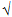(1-Mo^2): having angle of attack, not a, but/(1-Mo^2): and having its camber increased in the ratio
1 /(1-Mo^2).”

I can still remember the first time I read this. I was on the bus heading home from the reactor site. If only Nuclear Physics were as clear as this. All I had to do was find data for an airfoil stretched in 3 different directions and the job was done. I don’t think!

Next off the rank is my old mate Milne-Thompson in “Theoretical Aerodynamics”, who threw in a lot of mathematical chaff with the oats. Try this:

“Case I.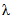= 1, v = 1. Here the chords are equal but the camber and thickness of A are those of Ai, reduced in the ratio ß:1. The lift, circulation, and pressure are the same at corresponding points in both flows. The incidence is reduced in the ratio ß:1.

Case II.= 1/ß, v = 1. Now c = ßci, so that the chord of A is less than the chord of Ai in the ratio ß:1 and the camber and thickness are reduced in the same ratio at corresponding points. The lift, shape, incidence and circulation are unaltered; the pressure is increased in the ratio 1:ß.

Case III.= 1, v = ß. In this case ß/ v = 1 and the distortions are the same in both flows. Thus A and Ai are identical profiles at the same incidence. The circulation, lift and pressure are increased in the ratio 1:ß. Thus the effect of compressibility on a given profile is to increase the lift in the ratio 1:ß.

End quote. That very last line is the clue. No more bending and twisting the airfoil profile. This is the same airfoil we started with. Compressibility changes its lift. Good old Milne-Thommo.

Perhaps I could have saved myself some trouble if I had understood Abbott and Von Doenhoff’s conttribbution.

“The Glauert-Prandtl rule relates the lift coefficient or slope of the lift curve of a wing section in compressible flow with that for incompressible flow”.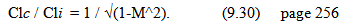With c meaning “compressible” and i meaning “incompressible”, this works. But I still can’t see how it works for the lift slope. Guess I’ll just have to stay thin and ignorant.